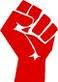Miscellaneous Quiz / Geometry Vocabulary

Random Miscellaneous or Definition Quiz

Can you name the geometry vocabulary?

byeweinmann  Plays Quiz not verified by Sporcle

Score
0/20
Timer
10:00
An unproven statement based on observations; a guess
A one-dimensional array of points with definite endpoints
A fancy geometry word for 'equal'
Two or more angles that add to 90 degrees
To cut into 2 equal parts
Two angles that are across from each other in an intersection of 2 lines
An angle measuring less than 90 degrees
An angle measuring 180 degrees
Two or more angles that add to 180 degrees
Two angles that are adjacent and together form a straight angle
An if-then statement
The negation of an if-then statement
The statement formed by negating AND switching the hypothesis and conclusion in an if-then statement
The property that states if G=H and H=J, then G=J
Two lines that do not intersect and are not coplanar
Two lines that do not intersect and are coplanar
Two lines that intersect to form a right angle
The point that is equidistant from both endpoints on a line segment
y - y1 = m(x - x1)
The basketball player on the poster in the back of Mr. Weinmann's classroom

From the Vault See Another

Country Words as Baby Names
Thankfully, not enough people have named their child Turkey to make this list.

Extras

Created Oct 6, 2009